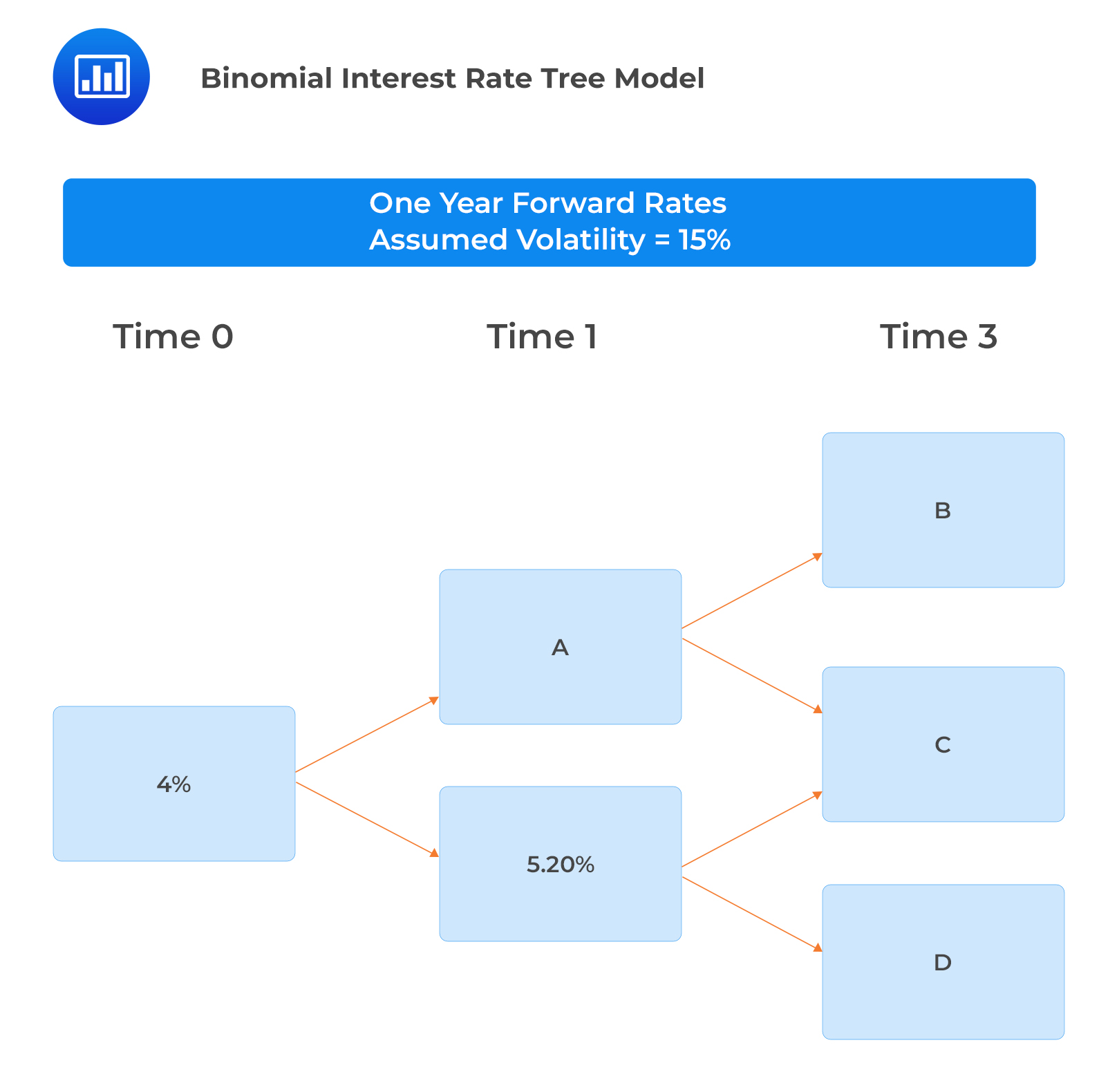Limited Time Offer: Save 10% on all 2022 Premium Study Packages with promo code: BLOG10# Calibrating a Binomial Interest Rate Tree

The following steps should be followed when calibrating binomial interest rate trees to match a particular term structure:

• Step 1: Estimate the appropriate spot and forward rates for a known par value curve.
• Step 2: Construct the interest rate tree using the assumed volatility and the interest rate model.
• Step 3: Determine the appropriate values for the zero-coupon bonds at each node using backward induction.
• Step 4: Calibrate the tree to ensure it is arbitrage-free. The value of a bond produced by the interest rate tree must be equal to its market price.

When constructing an interest rate tree as per step 2 above, it’s important to remember that:

• At each node, the forward interest rates can either go up (higher rate) or down (lower rate).
• You can determine the lower rate iteratively or by solving simultaneous equations depending on:
• The relationship: $$i_{1,d}=i_{1,u}\times e^{-2\sigma}$$.
• Known spot and forward rates.
• Features of the coupon bond, particularly its maturity.
• All this can be complicated to perform manually, but some Excel tools, such as Solver, can be employed to make it easy.
• Adjacent forward rates (for the same period) are two standard deviations apart. this means that if you know one of the forward rates for a particular nodal period, you can easily compute the other forward rates for that period in the tree.

The following related formulas are important to remember:

$$i_{1,u}=i_{1,d}e^{2\sigma }$$

$$i_{2,uu}=i_{2,dd}e^{4\sigma }$$

$$i_{2,uu}=i_{2,du}e^{2\sigma }$$

$$i_{2,du}=i_{2,dd}e^{2\sigma }$$

#### Example: Binomial Interest Rate Tree

To calibrate a binomial interest rate tree, a portfolio manager collects the following information relating to the spot rate curve and forward rates:

$$\begin{array}{c|c} \textbf{Term to Maturity} & \textbf{Spot Rate} \\ \hline 1 & 4.00\% \\ \hline 2 & 5.00\% \\ \hline 3 & 6.00\% \end{array}$$Determine the forward rates A, B, C, and D.

Forward rate A

\begin{align*} \text{The higher rate is determined as } i_{1u}&=i_{1d}\times e^{2\sigma} \\ &=5.20\%\times e^{2\times0.15}=7.019\% \end{align*}

Forward rate C

This is the middle forward rate approximated as $$f (2,1)$$. Recall from the previous reading the relationship between forward and spot rates:

\begin{align*} \left[1+f\left(t,T-t\right)\right]^{T-t} &=\left[\frac{\left(1+S_T\right)^T}{\left(1+S_t\right)^t}\right] \\ (1+f\left(2,1\right) &=\left[\frac{\left(1+S_3\right)^3}{\left(1+S_2\right)^2}\right] \\ f\left(2,1\right) &=\frac{{1.06}^3}{{1.05}^2}-1=8.029\% \end{align*}

Forward rate B

\begin{align*} i_{2,uu} &=i_{2du } e^{2\sigma} \\ i_{2,uu} &=8.029\%\times e^{2\times0.15 }=10.84\% \end{align*}

Forward rate D

\begin{align*} i_{2,dd} &=i_{2du} e^{-2\sigma} \\ i_{2,dd} &=8.029\%\times e^{-2\times0.15 }=5.95\% \end{align*}

It is key to note that the change in the volatility assumption affects implied forward rates. A change of volatility to a lower value makes the potential implied forward rates to collapse on the tree and vice versa.

## Question

Chen Cheng, a portfolio manager at ABC Investment Bank, is training Zhang Wang, a junior investment analyst, on calibrating binomial interest rate models using Excel. Cheng starts the process by guessing a lower one-year forward rate, $$i_{1,d}$$, of 3.820%. Assuming that Cheng uses a volatility assumption of 20%, the higher one-year forward rate using the lognormal model of interest rates is closest to:

1. 2.56%.
2. 4.67%.
3. 5.70%.

#### Solution

Based on the lognormal model of interest rates, the higher one-year forward rate is $$(i_{1,u})=i_{1,d}e^{2\sigma}$$.

\begin{align*} i_{1,d} &=3.820\% \\ (i_{1,u}) &=3.820\%\times e^{2\times0.20}=5.70\% \end{align*}

Reading 29: The Arbitrage-Free Valuation Framework

LOS 29 (d) Describe the process of calibrating a binomial interest rate tree to match a specific term structure.

Shop CFA® Exam Prep

Offered by AnalystPrepLevel I
Level II
Level III
All Three Levels
Featured Shop FRM® Exam PrepFRM Part I
FRM Part II
FRM Part I & Part II
Learn with Us

Subscribe to our newsletter and keep up with the latest and greatest tips for success
Shop Actuarial Exams PrepExam P (Probability)
Exam FM (Financial Mathematics)
Exams P & FM
Shop GMAT® Exam PrepComplete CourseDaniel Glyn
2021-03-24
I have finished my FRM1 thanks to AnalystPrep. And now using AnalystPrep for my FRM2 preparation. Professor Forjan is brilliant. He gives such good explanations and analogies. And more than anything makes learning fun. A big thank you to Analystprep and Professor Forjan. 5 stars all the way!michael walshe
2021-03-18
Professor James' videos are excellent for understanding the underlying theories behind financial engineering / financial analysis. The AnalystPrep videos were better than any of the others that I searched through on YouTube for providing a clear explanation of some concepts, such as Portfolio theory, CAPM, and Arbitrage Pricing theory. Watching these cleared up many of the unclarities I had in my head. Highly recommended.Nyka Smith
2021-02-18
Every concept is very well explained by Nilay Arun. kudos to you man!2021-02-13